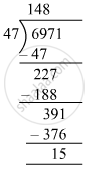# Divide and check your answer by the corresponding multiplication in the following: 39039 ​÷ 1001 - Mathematics

Fill in the Blanks

6971 ​÷ 47

#### SolutionQuotient = 148 and Remainder = 15
Check: Divisor × Quotient + Remainder = 47 × 148 + 15
= 6971
= Dividend
∴ Dividend = Divisor × Quotient + Remainder

Is there an error in this question or solution?

#### APPEARS IN

RS Aggarwal Class 6 Mathematics
Chapter 3 Whole Numbers
Exercise 3E | Q 2.1 | Page 56